﻿ How to Build an Temperature Sensor Circuit using an External LM34 IC with an STM32F407G Discovery Board in C ﻿# How to Build an Temperature Sensor Circuit using an External LM34 IC with an STM32F407G Discovery Board in CIn this article, we go over how to build a temperature sensor circuit using an external LM34 IC with an STM32F407G discovery board in C.

As a temperature sensor, the circuit will read the temperature of the surrounding environment and relay this temperature to us back in degrees fahrenheit.

The temperature sensor we will use is the LM34 IC, though you could use an LM35 IC.

The difference between an LM34 and a LM35 temperature sensor is the LM34 sensor gives out the temperature in degrees fahrenheit, while the LM35 sensor gives out the temperature in degrees celsius.

The IC we will use to measure the temperature in this circuit is the LM34 IC. We will integrate this with the arduino to measure the temperature. The arduino will then read this measured value from the LM34 and translate into degrees fahrenheit and celsius, which we will be able to read from the computer from the arduino serial monitor.

The LM34 is a low voltage IC which uses approximately +5VDC of power. This is ideal because the arduino's power pin gives out 5V of power. The IC has just 3 pins, 2 for the power supply and one for the analog output.

The output pin provides an analog voltage output that is linearly proportional to the fahrenheit temperature. Pin 2 gives an output of 1 millivolt per 0.1°F (10mV per degree). So to get the degree value in fahrenheit, all that must be done is to take the voltage output and divide it by 10- this gives out the value degrees in fahrenheit.

So, for example, if the output pin, pin 2, gives out a value of 785mV (0.785V), this is equivalent to a temperature of 78.5°F.

We can then easily convert this fahrenheit value into celsius by plugging in the appropriate conversion equation.

Below is the pinout of the LM34 IC: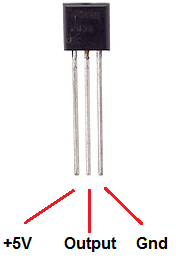Pin 1 receives positive DC voltage in order for the IC to work. This, again, is voltage approximately 5 volts. Pin 3 is the ground, so it receives the ground or negative terminal of the DC power supply. And Pin 2 is the output of the IC, outputting an analog voltage in porportion to the temperature it measures.

This is the datasheet of the LM34 IC: LM34 Temperature Sensor Datasheet.

The STM32F407G board, with suitable code, can then interpret this measured analog voltage and output to us the temperature in degrees celsius and fahrenheit.

We will connect our temperature sensor to pin PA1 (pin 1 of PORT A).

This is shown in the schematic diagram below.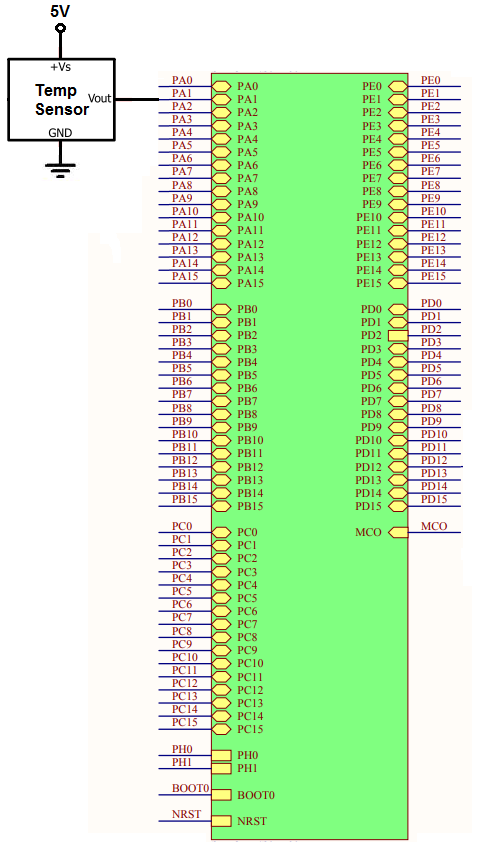We want to be able to get continuous samples from the temperature sensor, so we put the code in continuous conversions mode.

The header file we use that contains needed macros and register structures is shown below.

This file is named stm32f407.h

Its contents are shown below.

This header file contains the needed ADC register structure, as well as other items we need.

Below is the main.c file, which contains the functions we need to do an ADC conversion with continuous sampling from the temperature sensor.

So we have 3 include statements, which includes header files.

We create an integer, adc_value, which is the value returned from the sensor in our circuit.

Within our main() function, we initialize our ADC component with the pa1_adc_init() function.

We then start the conversion process with the adc_start_conversion() function.

We then have a while(1) function so that we create an infinite loop.

We read the value into our adc_value variable and then print out the value.

This is done continuously in an infinite loop.

Next, we go to our individual functions.

We have the function, pa1_adc_init(). This function initializes ADC functionality for pin PA1 of our board.

So in order to perform analog to digital conversion with an STM32F407G discovery board, we have to work with a variety of registers.

One of the first things to notice about all of the ADCs on the STM32F407G board is the bus that they are connected to it, which is the APB2 bus. This is important because we have to enable the peripheral clock of this bus in order to use the ADC component attached to it.

This diagram is shown below.You can see that all 3 ADCs are connected to this bus.

Thus, we must enable the clock of this bus.

This is done through the RCC APB2 peripheral clock enable register (RCC_APB2ENR), which is shown below.You can see on this register, that you can activate ADC1 by setting 1 to bit 8 activate ADC2 by setting 1 to bit 9, and activate ADC3 by setting 1 to bit 10.

On this register, you can see all of the rest of the components which are connected to the APB2 bus. This register is how you enable any component attached to this bus.

In our project, we will be working with ADC1, so we set 1 to pin 8.

So, with this now, we have activated the peripheral clock for component ADC1.

Next we need to choose a general I/O pin, which will function as an ADC pin. We need to then activate the peripheral clock register for this GPIO port.

We will choose pin 1 of GPIO port A. This is PA1.

All of the GPIO ports of the STM32F407G board are connected to the AHB1 bus. Therefore, in order to work with a GPIO pin, we need to enable the peripheral clock for the AHB1 bus.

You can see the diagram of this shown below.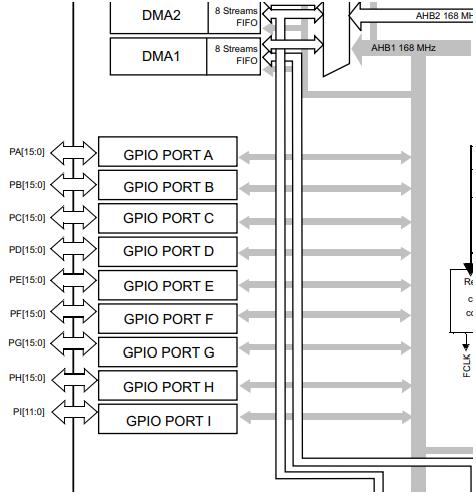So the next thing we have to do is look at the AHB1 peripheral clock register (RCC_AHB1ENR). This is shown below.So through this register, we can enable the peripheral clock for any GPIO port. You can see based on the diagram that pin 0 controls GPIO Port A, pin 1 controls GPIO Port B, pin 2 controls GPIO Port C, pin 3 controls GPIO Port D, pin 4 controls GPIO Port E, pin 5 controls GPIO Port F, pin 6 controls GPIO Port G, pin 7 controls GPIO Port I.

In this case, we want to use pin 1 of GPIO Port A, so we enable pin A.

We then must select the mode that the PA1 pin must be in. This is done through the GPIO port mode register (GPIOx_MODER).

This is shown in the diagram below.You can see that in order to make a pin analog, we need to write '11' to the pin. In the case of PA1, we need to write a 11 to pins 2 and 3, which is what we do in our code.

Next, we deal with ADC sampling.

The register we need to deal with in this case is the ADC regular sequence register 3 (ADC_SQR3).

This register is shown in the diagram below.SQ1, the first 4 bits of the register, is the first sequence of the ADC sampling. SQ2 would be if you have another ADC component. SQ3 would be if you have a third ADC component, and so on.

Since we are using ADC1 as the first (the only) sequence, we set bit 0 to 1.

The next thing we need to do is specify the sequence length (how many elements we are doing ADC sampling from).

This is done through the ADC regular sequence register 1. This is shown in the diagram below.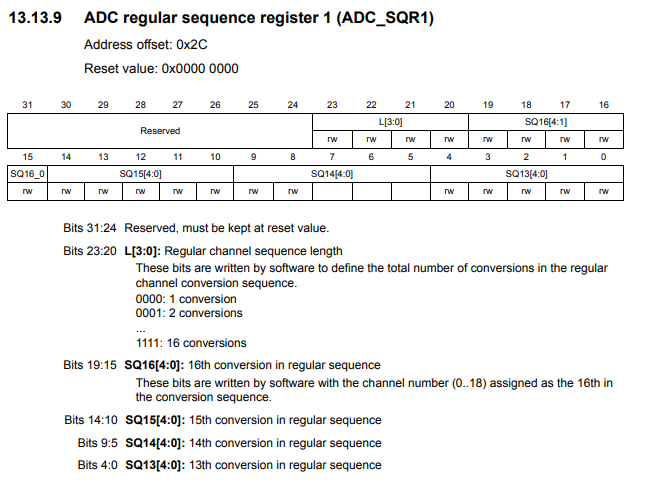Bits 20 to 23 control the regular channel sequence length. This determines the total number of conversions in the regular channel conversion sequence. In our case, it is 1. Therefore, we set bits 20 to 23 to 0. However, since everything should be 0, we simply set the entire register to 0.

The last thing we must do is enable the ADC, ADC1, in our case.

This is done through the ACD control register 2. This is shown in the diagram below.Bit 0 is the ADON bit, which stands for A/D converter ON/OFF. To enable the ADC, a 1 must be set to bit 0.

Next we go on to the next function, adc_start_conversion()

This function actually starts the ADC conversion cycle.

To start the conversion process, we use the ADC control register 2 (ADC_CR2), which is the register shown above.

Bit 30 is the SWSTART bit, which when set to 1 starts the conversion of regular channels.

To sample the value from the ADC component continuously, you would have to set the ADC in continuous mode.

Continuous mode is set by setting 1 to bit 1 of ADC control register 2.

We do this through the line, pADC1->CR2 |= (1 << 1);

This places the ADC in continuous sampling mode.

Before we can get the value of the ADC device, we must wait for the conversion to be complete. We use a while function to check for this. If the conversion is not complete, nothing is done. If the conversion is complete, we return the value from the ADC data register.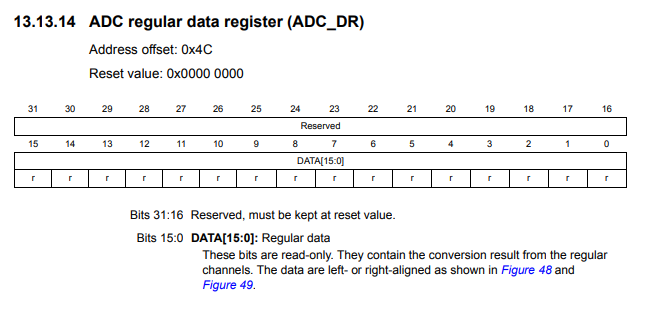The value that we get from the ADC regular data register is 12 bits, which means it returns values from 0 o 4095 (4096 data sampling points).

If we want to output the value in celsius rather than fahrenheit, we need to modify the adc_read() function so that the temperature value is output in celsius.

This is done below.

So if you replace the above adc_read() with this one, it will now output the temperature in degrees celsius.

And this is how to build a temperature sensor circuit using an external LM34 IC with an STM32F407G discovery board in C.

Related Resources

How to Set Bits of a Number in C

How to Clear Bits of a Number in C